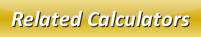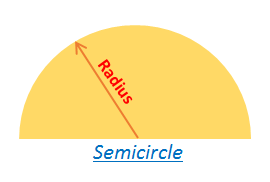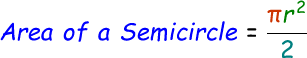# Area of a Semicircle Calculator

A semicircle is nothing but half of the circle. So the area of a semicircle is when you cut a full circle into half you get the area of a semicircle. We know the formula to calculate area of a circle is πr^2, by dividing this by 2 we will get the area of a semicircle.Therefore, the formula to calculate area of a semicircle is given by:where,
r = radius of the semicircle

In the below online area of a semicircle calculator, enter the radius in the input box and then click calculate button to find the answer.

 Radius of the Semicircle: Area of a Semicircle:

Latest Calculator Release

Average Acceleration Calculator

Average acceleration is the object's change in speed for a specific given time period. ...

Free Fall Calculator

When an object falls into the ground due to planet's own gravitational force is known a...

Torque Calculator

Torque is nothing but a rotational force. In other words, the amount of force applied t...

Average Force Calculator

Average force can be explained as the amount of force exerted by the body moving at giv...

Angular Displacement Calculator

Angular displacement is the angle at which an object moves on a circular path. It is de...